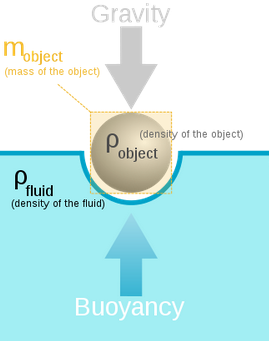#Buoyancy Mass Calculator

Calculates the buoyancy mass of the object by using object mass, fluid density and object density.## Buoyancy Object Mass

Buoyancy :

The upward force applied by the fluid on the object or the body when an object is put in or submerged in the fluid.## Buoyant Mass Formula:

mb = mo (1 - pfpo)

Where,

mb - buoyancy mass in kg

mo - true vaccum mass of the object in kg

pf - surrounding fluid density in kg/m3

po - object density in kg/m3